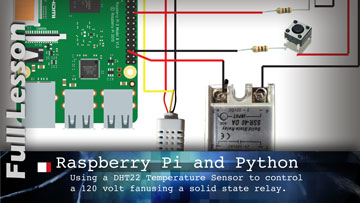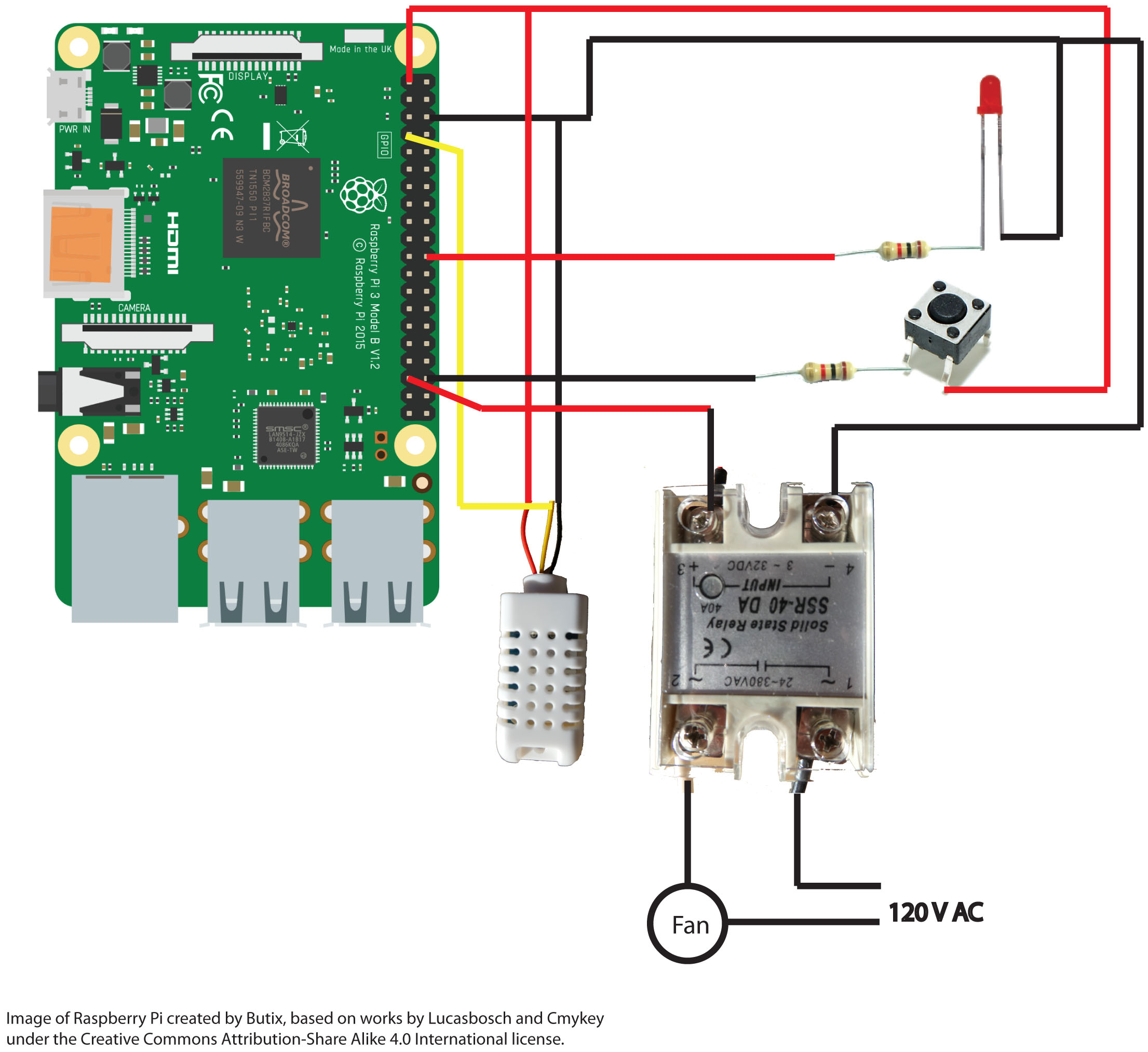# Raspberry Pi with Temperature and Moisture Sensor

## The Challenge

Create a system that will turn a fan on when the temperature in a greenhouse reaches a specific threshold temperature and off when it falls back below that threshold.

This project uses a Raspberry Pi 4, a BHT22 (AM2302) temperature and humidity sensor, and and SSR-40 DA solid state Relay to turn a 120V fan.

CAUTION: This project requires knowledge of working with 120V electricity. Do not attempt this without the proper training and experience.

## What you Need

• Raspberry Pi (3 or 4. 2 might also work)
• DHT22 (ASM2302) Temperature and Humidity Sensor
• SSR-40 DA solid state relay
• 40-pin Ribbon Cable (not required if you're not using a cobbler)
• T-Cobbler (optional)
• Push button
• LED
• 2 x 1k ohm resistors
• wires
• Receptable

Most parts are purchased from Elmwood Electronics https://elmwoodelectronics.ca/ Good prices and ship quickly.

If you're in the US, Adafruit https://www.adafruit.com/ is the source for so much maker wonderfulness.

## Hardware setup

The video covers the hardware setup but here are the main points.

The Raspberry Pi 4 connected to the breadboard by the ribbon cable and cobbler.

I’ve hooked the 3.3 volt power up to the power bus and the ground to the negative bus.

Then I connected the DHT22 sensor to power (red positive 3.3 V and black to ground) and the yellow wire to GPIO 4.

There is an LED that connects from the ground to a 1k resistor that connects to GPIO 25

The push button connects from the 3.3 V to a resistor and then GPIO 16.

SSR – Solid State Relay. GPIO 26 goes to the positive terminal of the 3.3V side and the negative terminal connects to the ground.## Source Code

'''
Fan turns on when temperature reaches threshold temperature or when button is pressed and
turns off when button is pressed or interval time ( in seconds) is reached.
Fan stays off for set interval if turned off when temperature is above threshold.
'''
import time
import RPi.GPIO as GPIO
from datetime import datetime
def round_up(n, decs=0):
# used for rounding up specified value n
import math
multiplier = 10 ** decs
return math.ceil(n * multiplier) / multiplier
def shut_down():
GPIO.output(led_pin, GPIO.LOW) # Turn off
print(datetime.now().strftime("%H:%M:%S")+" Shutting off Fan")
GPIO.setup(ssr_pin, GPIO.OUT, initial=GPIO.LOW) # Turn off 120V device
print(datetime.now().strftime("%H:%M:%S")+" Fan was on for: " + str(round(now - start_time, 1)) + " seconds")

def start_fan() :
GPIO.output(led_pin, GPIO.HIGH) # Turn on
GPIO.setup(ssr_pin, GPIO.OUT, initial=GPIO.HIGH) # Turn on 120V device
print(datetime.now().strftime("%H:%M:%S")+" Starting Fan at " + "Temp={0:0.1f}*C ".format(temperature))

def my_time():
#rounds up the time to the nearest second and returns an integer
#mytime=round_up(time.time())
mytime=round(time.time())
return int(mytime)

DHT_SENSOR = Adafruit_DHT.DHT22 # create a sensor object
##### Most common variables
on_interval = 10 # this is the interval (in seconds) the fan will stay on before checking to see if the temperature has dropped.
threshold_temp = 25   # the temperature that has to be reached before the fan starts
off_interval = 10 # if the fan is manually switched off, it will stay off for this period of seconds
##### Pins
DHT_PIN = 4 # specify the GPIO pin that the sensor yellow wire is connected to
led_pin = 25 # specify which GPIO the LED is connected to
ssr_pin = 26 # pin to trigger the relay
button_pin = 16 # pin that looks for the button being pressed
##### GPIO Setup
GPIO.setmode(GPIO.BCM)   # Use Broadcom GPIO numbering not physical numbering
GPIO.setwarnings(False)    # Ignore warning for now
GPIO.setup(led_pin, GPIO.OUT, initial=GPIO.LOW) # LED output
GPIO.setup(ssr_pin, GPIO.OUT, initial=GPIO.LOW) # SSR output
GPIO.setup(button_pin, GPIO.IN, pull_up_down=GPIO.PUD_DOWN) # connected to push button
##### Variable initialization
start_time = int() # the time when the temperature first went above the threshold or the button was pressed
init_time = int()  # the time the program was started
off_time = int()   # the time that the fan was shut off
last_check = int() # the time that the temperature was last checked.
now = int()        # the time right now
init_time = my_time()
timer = int() # counts seconds that the system is running

if humidity is not None and temperature is not None:
print(datetime.now().strftime("%H:%M:%S") + " Temp={0:0.1f}*C  Humidity={1:0.1f}%".format(temperature, humidity))
else:
print(atetime.now().strftime("%H:%M:%S") + "Failed")

while True:
now = my_time()
# fan is not running and it was not switched off
if GPIO.input(ssr_pin) == 0  and off_time == 0:
if (temperature >= threshold_temp) or GPIO.event_detected(16): #threshold temperature reached or button is pressed
start_fan()
start_time = my_time()
# Fan is running and button pressed
elif GPIO.input(ssr_pin) == 1 and GPIO.event_detected(16):
now = my_time()
off_time = now
shut_down() # shut down fan
# Fan is running and time interval exceeded
elif GPIO.input(ssr_pin) == 1 and now - start_time > on_interval:
if temperature < threshold_temp: # temp falls below threshold
now = my_time()
shut_down() # shut down fan
else: # temp is still above threshold but the on interval has been exceeded
start_time = now
# fan is off because it was switched off. Need to keep it off.
elif GPIO.input(ssr_pin) == 0 and off_time > 0:
if now - off_time > off_interval:
print (datetime.now().strftime("%H:%M:%S")+" Fan was off for: " + str(now - off_time) + " seconds")
off_time = 0
if (now-init_time) % 3 == 0: # If the current number of seconds since the system started is even ie: a 3-second interval
print(datetime.now().strftime("%H:%M:%S ") + "Temp={0:0.1f}*C  Humidity={1:0.1f}%".format(temperature, humidity))
GPIO.cleanup()